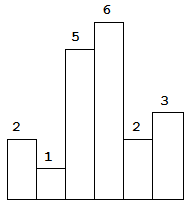# Leetcode 84 求直方图中矩形的最大面积

## 题目描述

Leetcode 84 给定 n 个正整数的列表，表示矩形的高度，表示直方图。每一个给出的矩形宽度是 1，找到在直方图里最大的矩形面积。Input: [2,1,5,6,2,3]
Output: 10


## 题目分析

class Solution:
def largestRectangleArea_old(self, heights: List[int]) -> int:
"""
O(n^2) -> 超时了，无法提交成功
:param heights:
:return:

思路：最后形成最大的矩形面积，肯定是以给定的数组中某个位置连续矩形的最矮位置。
从 index=0 的位置开始，假定它是这样的位置，然后向左向右开始扫描，找出其连续的矩形
面积，然后依次比较这些找到的矩形面积。
"""
max_area = 0
index = 0
list_length = len(heights)
while index < list_length:
area = heights[index]
left_index = index - 1
while left_index > -1:
if heights[left_index] >= heights[index]:
area += heights[index]
left_index -= 1
else:
break

right_index = index + 1
while right_index < list_length:
if heights[right_index] >= heights[index]:
area += heights[index]
right_index += 1
else:
break
max_area = max_area if max_area > area else area
index += 1

return max_area


• 给定的元素可能是一直递增的，所以需要给 heights 末尾添加一个元素 0，用于计算之前的面积。
• 需要考虑出栈后，站内元素为 0 的情况，这时需要计算，最低矩形高度可以形成的连续矩形面积的情况。例如 [2,1,2] 这种情况。
class Solution:
def largestRectangleArea(self, heights: List[int]) -> int:
# add 0 to query the last local peak area
# if the last height is still the highest
heights.append(0)
# definite a stack to record the heights position
# to get the local peak area
heights_position = []
max_area = 0
#
index = 0
while index < len(heights):
if len(heights_position) == 0 or heights[heights_position[-1]] <= heights[index]:
heights_position.append(index)
index += 1
else:
popped_position = heights_position.pop()
# get the continuous area of the smallest rectangle
# index represents the the number of elements has been processed
if len(heights_position) == 0:
max_area = max(max_area, index * heights[popped_position])
# Get maximum area of rectangle in monotonically increasing
else:
# index need to reduce 1 because the we add a 0
#  to the end of heights array.
max_area = max(max_area,
(index - 1 - heights_position[-1]) * heights[popped_position])
return max_area


posted @ 2019-12-26 11:04  以终为始  阅读(...)  评论(...编辑  收藏